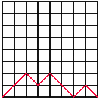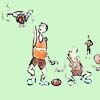# Search by Topic

#### Resources tagged with Patterned numbers similar to Tree Graphs:

Filter by: Content type:
Age range:
Challenge level:

### There are 19 results

Broad Topics > Numbers and the Number System > Patterned numbers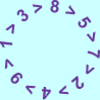### Whole Number Dynamics II

##### Age 14 to 18

This article extends the discussions in "Whole number dynamics I". Continuing the proof that, for all starting points, the Happy Number sequence goes into a loop or homes in on a fixed point.### Whole Number Dynamics III

##### Age 14 to 18

In this third of five articles we prove that whatever whole number we start with for the Happy Number sequence we will always end up with some set of numbers being repeated over and over again.### Whole Number Dynamics IV

##### Age 14 to 18

Start with any whole number N, write N as a multiple of 10 plus a remainder R and produce a new whole number N'. Repeat. What happens?### Whole Number Dynamics V

##### Age 14 to 18

The final of five articles which containe the proof of why the sequence introduced in article IV either reaches the fixed point 0 or the sequence enters a repeating cycle of four values.### Magic Squares II

##### Age 14 to 18

An article which gives an account of some properties of magic squares.### Whole Number Dynamics I

##### Age 14 to 18

The first of five articles concentrating on whole number dynamics, ideas of general dynamical systems are introduced and seen in concrete cases.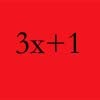### Try to Win

##### Age 16 to 18

Solve this famous unsolved problem and win a prize. Take a positive integer N. If even, divide by 2; if odd, multiply by 3 and add 1. Iterate. Prove that the sequence always goes to 4,2,1,4,2,1...### Harmonic Triangle

##### Age 14 to 16 Challenge Level:

Can you see how to build a harmonic triangle? Can you work out the next two rows?### Pinned Squares

##### Age 14 to 16 Challenge Level:

What is the total number of squares that can be made on a 5 by 5 geoboard?### Back to Basics

##### Age 14 to 16 Challenge Level:

Find b where 3723(base 10) = 123(base b).### Odd Differences

##### Age 14 to 16 Challenge Level:

The diagram illustrates the formula: 1 + 3 + 5 + ... + (2n - 1) = n² Use the diagram to show that any odd number is the difference of two squares.### Magic Squares

##### Age 14 to 18

An account of some magic squares and their properties and and how to construct them for yourself.### Triangles Within Pentagons

##### Age 14 to 16 Challenge Level:

Show that all pentagonal numbers are one third of a triangular number.### How Old Am I?

##### Age 14 to 16 Challenge Level:

In 15 years' time my age will be the square of my age 15 years ago. Can you work out my age, and when I had other special birthdays?### Sixty-seven Squared

##### Age 16 to 18 Challenge Level:

Evaluate these powers of 67. What do you notice? Can you convince someone what the answer would be to (a million sixes followed by a 7) squared?### Rolling Coins

##### Age 14 to 16 Challenge Level:

A blue coin rolls round two yellow coins which touch. The coins are the same size. How many revolutions does the blue coin make when it rolls all the way round the yellow coins? Investigate for a. . . .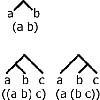### Counting Binary Ops

##### Age 14 to 16 Challenge Level:

How many ways can the terms in an ordered list be combined by repeating a single binary operation. Show that for 4 terms there are 5 cases and find the number of cases for 5 terms and 6 terms.